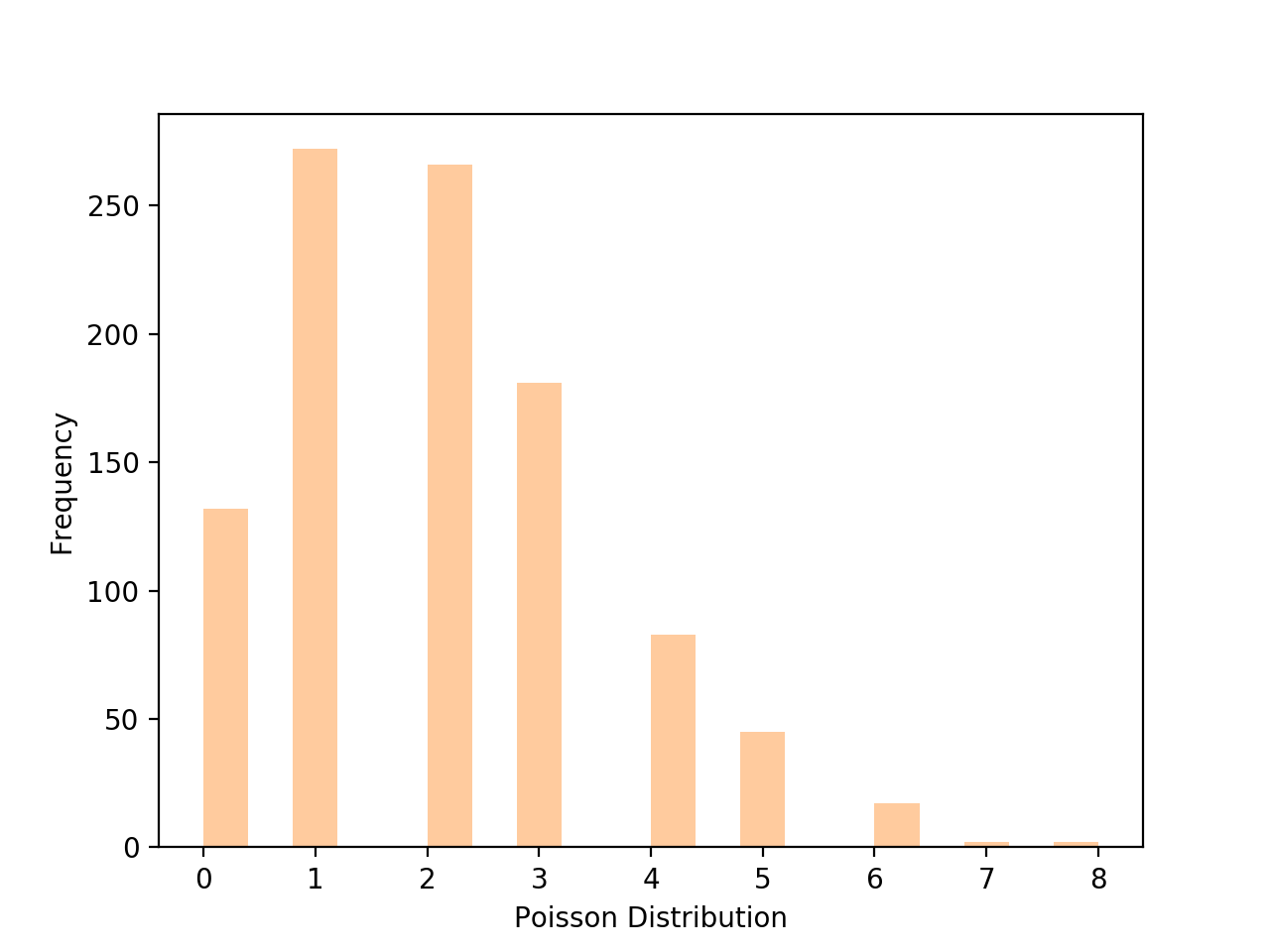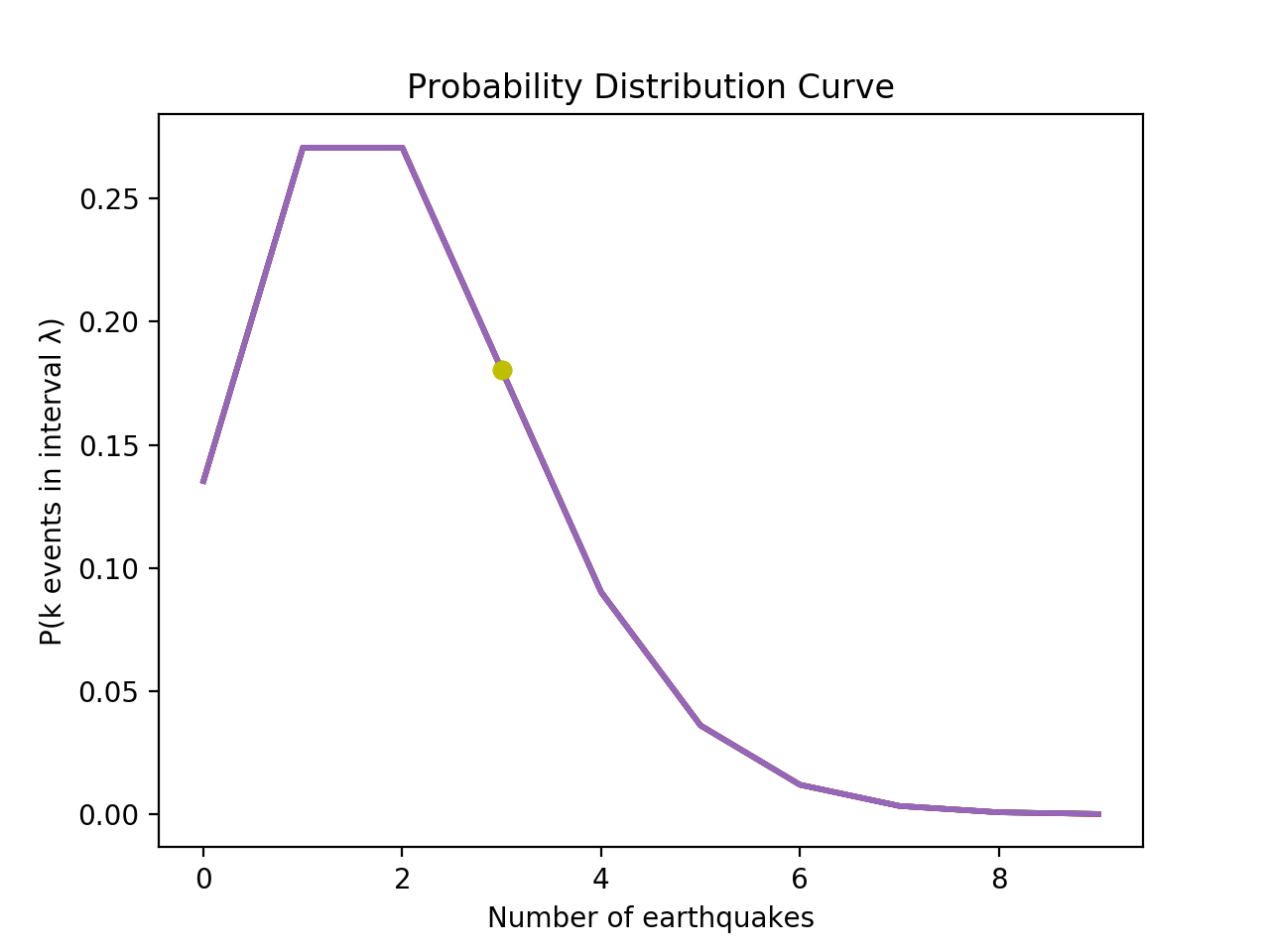If you’re just getting started with data analytics, you’ll be getting to grips with some relatively complex statistical concepts. One such concept is probability distribution—a mathematical function that tells us the probabilities of occurrence of different possible outcomes in an experiment. There are six main types of distribution, but today we’ll be focusing on just one: the Poisson distribution.

By the end of this post, you’ll have a clear understanding of what the Poisson distribution is and what it’s used for in data analytics and data science. We’ve divided our guide as follows:

So, what exactly is a Poisson distribution? Allow me to explain!

## 1. What is the Poisson process?

Before we talk about the Poisson distribution itself and its applications, let’s first introduce the Poisson process. In short, the Poisson process is a model for a series of discrete events where the average time between events is known, but the exact timing of events is random. The occurrence of an event is also purely independent of the one that happened before.

So let’s bring this theory to life with a real-world example. We all get frustrated when our internet connection is unstable. If we assume that one failure doesn’t influence the probability of the next one, we might say that it follows the Poisson process, where the event in question is “internet failure”. All we need to know is the average time between these failures. However, there is a set of criteria that needs to be met:

1. The events of such a process are independent of each other.
2. The average rate of event occurrences per unit of time (e.g. per month) is constant.
3. Two events (e.g. internet failure or no internet failure) cannot occur simultaneously.

In our internet example, we assume that the events are independent and unrelated; that is, one instance of internet failure doesn’t affect the probability of the next instance. But sometimes, this might not be the case.

Another frequently given example for a Poisson process is Uber arrivals. However, this is not a true Poisson process because the arrivals are not completely independent of one another. Even for buses that do not run on time, we cannot be sure that their late arrival doesn’t affect the arrival time of the next bus.

On the other hand, cases such as customers calling a help center or visitors landing on a website are more likely to be independent and would probably be considered a more solid example of the Poisson process.

## 2. What is the Poisson distribution?

While the Poisson process is the model we use to describe events that occur independently of each other, the Poisson distribution allows us to turn these “descriptions” into meaningful insights. So, let’s now explain exactly what the Poisson distribution is.

### The Poisson distribution is a discrete probability distribution

As you might have already guessed, the Poisson distribution is a discrete probability distribution which indicates how many times an event is likely to occur within a specific time period. But what is a discrete probability distribution?

Right, let’s first align on the concepts! A probability distribution is a mathematical function that gives the probabilities of possible outcomes happening in an experiment. As you might already know, probability distributions are used to define different types of random variables. These variables can be either discrete or continuous. When talking about Poisson distribution, we’re looking at discrete variables, which may take on only a countable number of distinct values, such as internet failures (to go back to our earlier example).

Given all that, Poisson distribution is used to model a discrete random variable, which we can represent by the letter “k”. As in the Poisson process, our Poisson distribution only applies to independent events which occur at a consistent rate within a period of time. In other words, this distribution can be used to estimate the probability of something happening a certain amount of times based on its event rate.

For example, if the average number of people who visit an exhibition on Saturday evening is 210, we can ask ourselves a question like “What is the probability that 300 people will visit the exhibition next week?”

### Getting hands-on with Poisson distribution

So far, we’ve covered lots of theory. Now it’s time to delve into the mathematical side of Poisson distribution.

First, let’s consider the formula used to calculate our probabilities. Discrete probability distributions are defined by probability mass functions, also referred to as pmf. In statistics, a probability mass function is a function that gives you the probability that a discrete random variable (i.e., “k”) is exactly equal to some value. So, Poisson distribution pmf with a discrete random variable “k” is written as follows:Hang on, don’t run away just yet! Let’s break it down:

• P(k events in interval) stands for “the probability of observing k events in a given interval”; that’s what we’re trying to find out.

• ” e “ is the Euler’s number, which is a mathematical constant with an approximate value of 2.71828.

• ” λ “ represents lambda, which is the expected number of possible occurrences. It is also sometimes called the rate parameter or event rate, and is calculated as follows: events/time * time period.

•  ” ! “ is the symbol used to represent the factorial function. Factorials are products of each whole number from 1 to k. So, in terms of the formula above, the factorial function tells us to multiply all whole numbers from our chosen number down to 1. For example, if “k” is 4, “k!” essentially means: 4! = 1 * 2 * 3 * 4. So, k! = 24.

To get a better grasp of how it works, let’s apply the formula to the following example.

The average number of internet failures in a household is 2 per week (“λ”). What is the probability of 3 (“k”) internet failures happening next week? Assuming that these are independent events with a constant average event rate and that can’t happen simultaneously, let’s fill in the data we have:

P (k; λ) = e-λ  *  λk  / k!

= 2.71828 – 2 * 23 / 3!

= 0.13534 * 8 / 6

≈ 0.18

Seems like the probability of 3 internet failures happening next week is around 18%, which is not that high.

Calculating formulas manually can be a rather tedious process, and, as a data analyst or a data scientist, it’s highly unlikely that you’ll ever do it as we have above! There are certain tools and computer languages that enable you to analyze your data without having to go through such formulas manually.

One such language is Python, a programming language which is used to create algorithms (or sets of instructions) that can be read and implemented by a computer. We won’t go into detail about Python here; for the purpose of this post, you just need to know that it can be used to simplify the process of calculating a Poisson distribution for a given set of data. If you’d like to learn more about what Python is, we’ve covered it in detail here: What is Python? A Complete Guide.

With that in mind, we’re now going to do the following:

1. Generate some random Poisson-distributed data with Python
2. Visualize our data

### Generating and visualizing a Poisson distribution with Python

Below, you’ll see a snippet of code which will allow you to generate a Poisson distribution with the provided parameters (mu or also λ and size). In the code snippet itself, you’ll find explanations after the # sign, which is the way we do it in Python.

You can run this code either in your shell after installing Python to your local machine or simply by using the built-in shell at the official Python website.

Now let’s consider how our Poisson distribution might look in visual form. We can plot our data using seaborn, a Python data visualization library based on matplotlib. You can learn more about Python’s various libraries and what they’re used for in this guide.Here we can see the frequencies of an internet failure happening with event rate λ = 2.

We can also draw the probabilities. Below we see the probabilities of internet failures happening during the week. As we have already calculated, the probability of 3 internet failures happening next week is only 18%.In case you would like to generate your own probability plot and experiment with values and plot parameters, here is the code block below. If you find it difficult to follow, as usual, just check out the comments starting with “ # ”.

## 3. What is the Poisson distribution used for?

Now we know what the Poisson distribution is and what it looks like in action, it’s time to zoom out again and see where the Poisson distribution fits into the bigger picture.

As you know, data analytics is all about drawing meaningful insights from raw data; insights which can be used to make smart decisions. Poisson distributions are commonly used to find the probability that an event might happen a specific amount of times based on how often it usually occurs. Based on these insights and future predictions, organizations can plan accordingly.

For example, an insurance company might use Poisson distribution to calculate the probability of a number of car accidents happening in the next six months, which in turn will inform how they price the cost of car insurance.

Likewise, a call center might use Poisson distribution to predict how many incoming calls they’re most likely to receive throughout the week based on an already known event rate. This could help them to decide how many people to employ for the call center, or how many hours to allocate to each employee.

As you can see, the Poisson distribution has many real-world uses, making it an important part of the data analyst’s toolkit.

## 4. Key takeaways

We have now covered a complete introduction to the Poisson distribution. There is certainly a lot more to be explored and plenty more exciting problems to solve, but hopefully this has given you a good starting point from which to continue your journey of discovery!

Before we finish, let’s summarize the main properties of Poisson distribution and the key takeaways from what we’ve covered:

• Poisson distributions are used to find the probability that an event might happen a definite number of times based on how often it usually occurs.
• The average number of outcomes per specific time interval is represented by λ and is called an event rate.
• The events are independent, meaning the number of events that occur in any interval of time is independent of the number of events that occur in any other interval.
• The probability of an event is proportional to the length of time in question (e.g. a week or a month).
• The probability of an event in a particular time duration is the same for all equivalent time durations.

To learn more about Poisson distribution and its application in Python, I can recommend Will Koehrsen’s use of the Poisson process to simulate impacts of near-Earth asteroids. For a hands-on introduction to the field of data in general, it’s also worth trying out this free five-day data analytics short course. And, if you’d like to learn more about the techniques and tools used by data analysts, check out the following:

### What You Should Do Now

1. Get a hands-on introduction to data analytics with a free, 5-day data analytics short course.
2. Take a deeper dive into the world of data analytics with our Intro to Data Analytics Course.
3. Talk to a program advisor to discuss career change and find out if data analytics is right for you.
4. Learn about our graduates, see their portfolio projects, and find out where they’re at now.